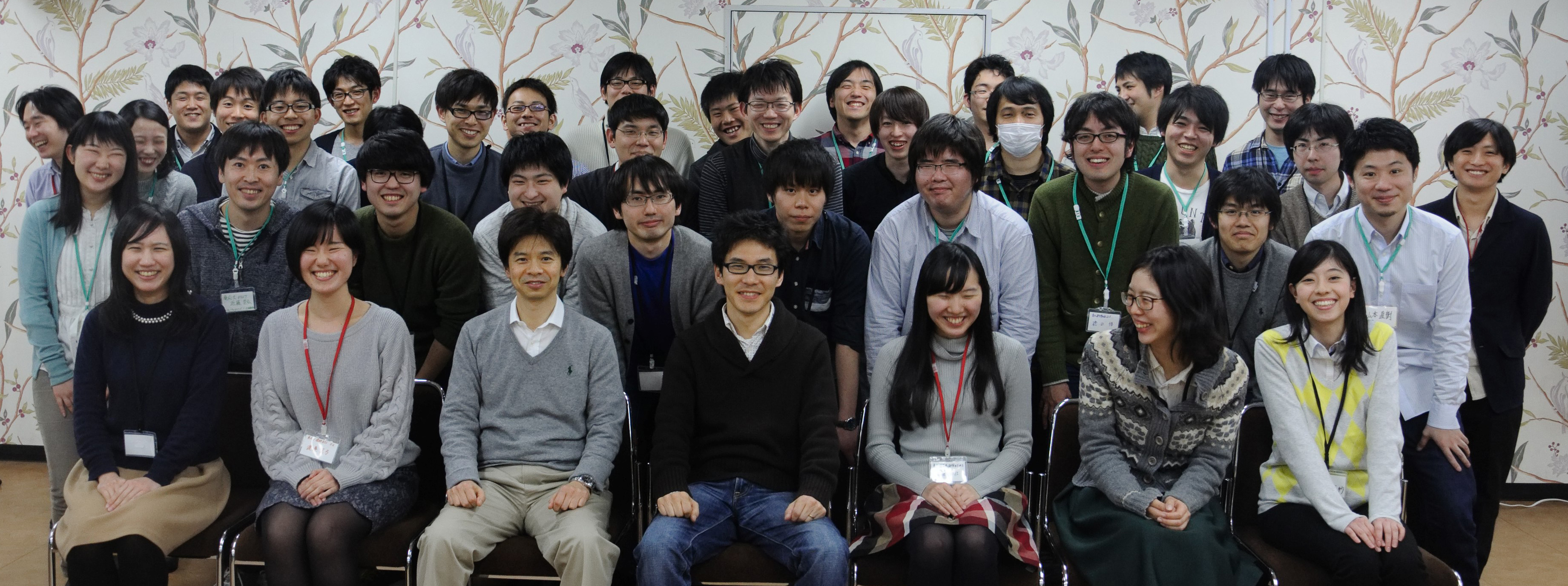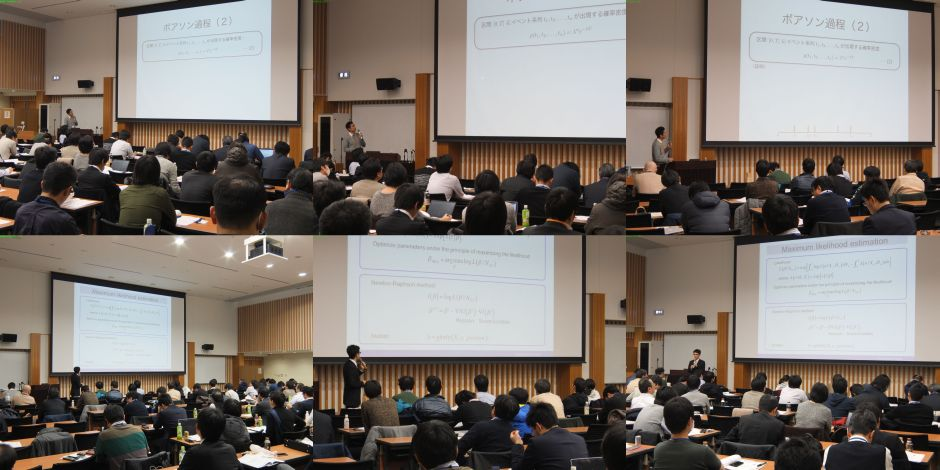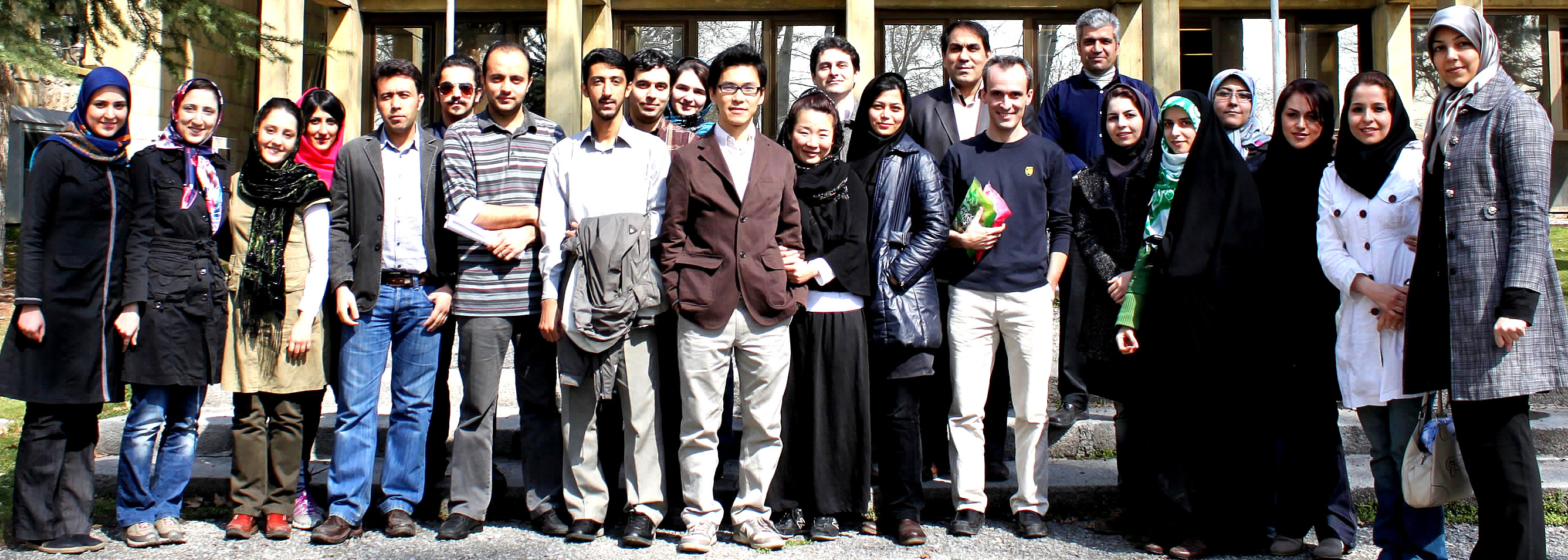Lecture slides Lecture slides on statistical modeling

 Lecture slides - Classical statistics - Statistical modeling - Point process Links - Histogram Optimization - Kernel Optimization - State-space analysis - HOME Page

# Classical statistics lectures

Slides of lectures given at RIKEN BSI and Kyoto University.
Slides for statistical tests are joint work of Kentaro Katahira and Hideaki Shimazaki.

# Statistical modeling lectures

Slides of lectures given at Kyoto University

# Point process theory

## Lecture 5: State-space model and a point process filter (Decoding)

#### Lectures on point process thery

2017 Mar 4 Brain wakate2016 Feb 10 Introduction to the point process networkA photo cited from the lecture page of Institute of Statistics and Mathematics. http://www.ism.ac.jp/lectures/27n.html

2013 Mar 3-12 Introduction to statistical models of neural spike train data [link]

Two-weeks course at the Institute for Research in Fundamental Sciences, School of Cognitive Sciences